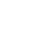Introducing Visual Explorer, a new tool for data visualization.Learn more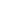# Forecasting in Python with Prophet

Forecasting is often considered a natural progression from reporting. Reporting helps us answer, what happened? Forecasting helps answer the next logical question, what will happen?

Historically, high quality forecasts have been very challenging to produce. This resulted in a severe shortage of analysts who could deliver forecasts with the level of accuracy required to drive business decisions. To alleviate this supply gap and to make scalable forecasting dramatically easier, the Core Data Science team at Facebook created Prophet, a forecasting library for Python and R, which they open-sourced in 2017.

The intent behind Prophet is to “make it easier for experts and non-experts to make high-quality forecasts that keep up with demand.” Prophet is able to produce reliable and robust forecasts (often performing better than other common forecasting techniques) with very little manual effort, while allowing for the application of domain knowledge via easily-interpretable parameters.

In this recipe, you'll learn how to use Prophet (in Python) to solve a common problem: forecasting a company's daily orders for the next year. This lightweight example should serve as a great way to get started with Prophet, and will hopefully spark some inspiration to dive even deeper into the library's vast functionality.

This recipe is broken down into four main sections:

You can find implementations of all of the steps outlined below in this example Mode report.

### Data Preparation & Exploration

Prophet works best with daily periodicity data with at least one year of historical data. It's possible to use Prophet to forecast using sub-daily or monthly data, but for the purposes of this recipe, we'll use the recommended daily periodicity. We will use SQL to wrangle the data we’d like to forecast at a daily periodicity:

``````select
date,
value
from modeanalytics.daily_orders
order by date
``````

NOTE: While Prophet is relatively robust to missing data, it’s important to ensure that your time series is not missing a significant number of observations. If your time series is missing a large number of observations, consider using a resampling technique or forecasting your data at a lower frequency (e.g. making monthly forecasts using monthly observations)

Now that we have our data at a daily periodicity, we can pipe our SQL query result set into a pandas dataframe object in the Python notebook. First, rename your SQL query to `Daily Orders`. Then, inside the Python notebook, we can use the following statement to pipe our query result set into a dataframe `df`:

``````df = datasets["Daily Orders"]
``````

To get a quick sense of how many observations your dataframe contains, you can run the following statement, which will return a tuple containing the number of rows and columns in your dataframe, respectively:

``````df.shape
``````

Prophet always expects two columns in the input DataFrame: `ds` and `y`. The `ds` column represents the date from your SQL query, and needs to be either date or datetime data type. The `y` column represents the value we are looking to forecast, and must be of numeric data type. To check the types of the columns in your DataFrame, you can run the following statement in the Python notebook:

``````df.dtypes
``````

Once you have confirmed that the columns in your dataframe are the correct data types, you can create a new column `ds` in your dataframe that is an exact copy of the `date` column, and a new column `y` that is an exact copy of the `value` column:

``````df['ds'] = df['date']
df['y'] = df['value']
``````

You can then repurpose the `date` column to be used as the index of the dataframe:

``````df.set_index('date')
``````

This transforms the index of your dataframe into a DatetimeIndex, which gives pandas the capability to interpret this dataset as a Time Series.

Now that you have your data prepped to be used with Prophet, it’s good practice to plot it and inspect what the data looks like before feeding it into Prophet. Using our example data, we can use matplotlib to create the following visualization: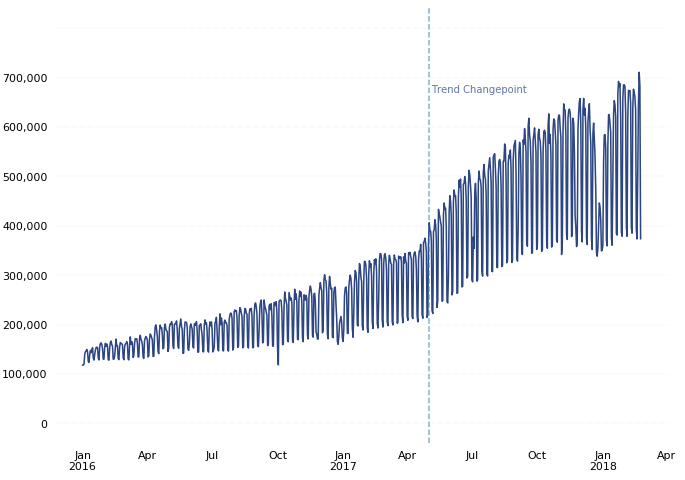There are a few things to notice about this plot:

1. There is a noticeable change in trend trajectory around May 2017. By default, Prophet automatically detects these kinds of “trend changepoints” and allows the trend to adapt appropriately. Prophet also allows finer-grained control over the identification of these trend changepoints.
2. There is noticeable weekly and yearly seasonality. Prophet will automatically fit weekly and yearly seasonalities if the time series is more than two cycles long.
3. The mean and variance of our observations increase over time. Prophet natively models the increase in mean of the data over time, but we should take additional steps to normalize as much variance as possible to achieve the most accurate forecasting results. We can do this by applying a power transform to our data.

### Box-Cox Transform

Often in forecasting, you'll explicitly choose a specific type of power transform to apply to the data to remove noise before feeding the data into a forecasting model (e.g. a log transform or square root transform, amongst others). However, it can sometimes be difficult to determine which type of power transform is appropriate for your data. This is where the Box-Cox Transform comes in.

Box-Cox Transforms are data transformations that evaluate a set of lambda coefficients (λ) and selects the value that achieves the best approximation of normality.

The scipy.stats Python module provides a built-in Box-Cox Transform method, called boxcox. The `boxcox` method returns a positive dataset transformed by a Box-Cox power transformation. To use the `boxcox` method, first import the method from the `scipy.stats` module by adding the following line to your import block:

``````from scipy.stats import boxcox
``````

The `boxcox` method has one required input: a 1-dimensional array of positive data to transform. Optionally, you can also specify the λ value you’d like to use for your transformation (e.g. λ = 0 for a log transform). Otherwise, the `boxcox` method will find the λ that maximizes the log-likelihood function and will return it as the second output argument.

For our example, we will let the `boxcox` method determine the optimal λ to use for our transformation, and will return that value to a variable named lam:

``````# Apply Box-Cox Transform to value column and assign to new column y
df['y'], lam = boxcox(df['value'])
``````

If we plot our newly transformed data alongside the untransformed data, we can see that the Box-Cox transformation was able to remove much of the increasing variance in our observations over time: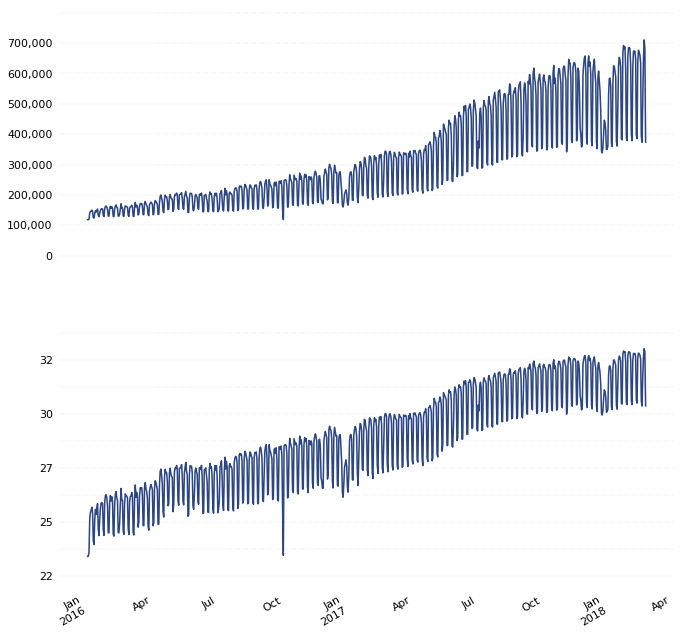### Forecasting

The first step in creating a forecast using Prophet is importing the `fbprophet` library into our Python notebook:

``````import fbprophet
``````

Once we've imported the Prophet library into our notebook, we can begin by instantiating (create an instance of) a Prophet object:

``````m = fbprophet.Prophet()
``````

Once you have instantiated a Prophet object, you're ready to fit a model to your historical data. You do this by calling the `fit` method on the Prophet object and passing in your dataframe:

``````m.fit(df)
``````

Once you have used Prophet to fit the model using the Box-Cox transformed dataset, you can now start making predictions for future dates. Prophet has a built-in helper function `make_future_dataframe` to create a dataframe of future dates. The `make_future_dataframe` function lets you specify the frequency and number of periods you would like to forecast into the future. By default, the frequency is set to days. Since we are using daily periodicity data in this example, we will leave `freq` at it’s default and set the `periods` argument to 365, indicating that we would like to forecast 365 days into the future.

``````future = m.make_future_dataframe(periods=365)
``````

We can now use the `predict` method to make predictions for each row in the future dataframe.

``````forecast = m.predict(future)
``````

At this point, Prophet will have created a new dataframe assigned to the `forecast` variable that contains the forecasted values for future dates under the column `yhat`, as well as uncertainty intervals and components for the forecast. We can visualize the forecast using Prophet’s built-in `plot` helper function:

``````m.plot(forecast);
``````

In our example, our forecast looks as follows: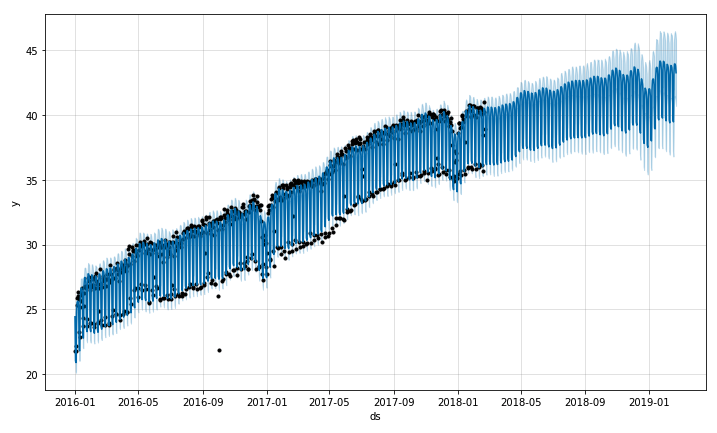If you want to visualize the individual forecast components, you can use Prophet’s built-in `plot_components` method:

``````m.plot_components(forecast);
``````

Running `plot_components` on our example data returns the following set of component visualizations: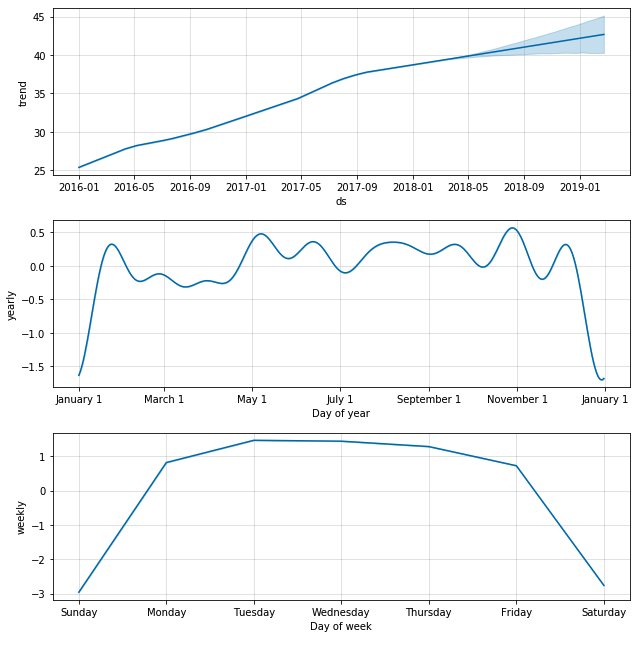The forecast and component visualizations show that Prophet was able to accurately model the underlying trend in the data, while also accurately modeling weekly and yearly seasonality (e.g. lower order volume on weekend and holidays).

### Inverse Box-Cox Transform

Since Prophet was used on the Box-Cox transformed data, you'll need to transform your forecasted values back to their original units. To transform your new forecasted values back to their original units, you will need to perform an inverse Box-Cox transform.

The scipy.special Python module provides a built-in Inverse Box-Cox Transform method, called inv_boxcox. To use the `inv_boxcox` method, first import the method from the `scipy.special` module by adding the following line to your import block:

``````from scipy.special import inv_boxcox
``````

The `inv_boxcox` method has two required inputs; an array of data to transform and a λ value for the transform. We will inverse transform specific columns in our forecast dataframe, and supply the λ value we obtained earlier from our first Box-Cox transform stored in the `lam` variable:

``````# Apply inverse Box-Cox transform to specific forecast columns
forecast[['yhat','yhat_upper','yhat_lower']] = forecast[['yhat','yhat_upper','yhat_lower']].apply(lambda x: inv_boxcox(x, lam))
``````

Now that your forecasted values are transformed back to their original units, you are able to visualize the forecasted values alongside the historical values: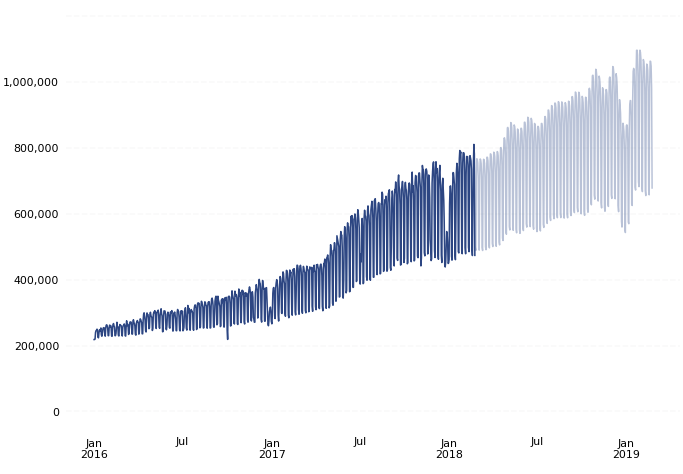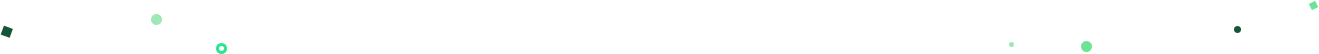# Get our weekly data newsletter

Work-related distractions for every data enthusiast.